• 众所周知，二分类问题的损失函数为： 其中y代表标签值（0,1）；h(θ,x)代表通过假设假设函数（sigmoid 函数）计算出的函数值（概率），sigmoid 函数的取值区间为（0,1） 当标签值为1时，h(θ,x)的值为预测y...
二分类

众所周知，二分类问题的损失函数为：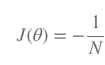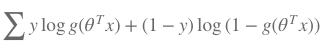其中y代表标签值（0,1）；h(θ,x)代表通过假设假设函数（sigmoid 函数）计算出的函数值（概率），sigmoid 函数的取值区间为（0,1）当标签值为1时，h(θ,x)的值为预测y为1的概率，这个值越靠近0，logh(θ,x)的值就会越大，从而对loss值的惩罚也就越大；反之当标签值为0时，1-h(θ,x)的值为预测y为0的概率，这个值越靠近0，log(1-h(θ,x))的值就会越大。

二分类问题的决策函数为：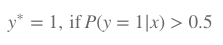多分类（softmax)

二分类问题也可以看做是多分类问题的特例，由此，K分类问题的loss函数表达式也可以延伸为: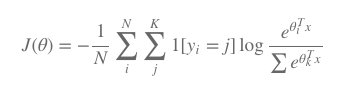多分类问题（softmax）的决策函数为：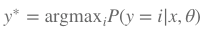（与二分类函数的决策函数比较，二分类函数的决策函数依然可以视为K分类问题的特例）

总结

由此不难看出，在keras里面activation选择sigmoid的时候，与之对应的loss为binary_crossentropy；同理，activation选择softmax的时候，与之对应的loss为categorical_crossentropy

参考资料

https://tech.meituan.com/intro_to_logistic_regression.html

展开全文• 目标：求出逻辑回归损失函数的最小值。 逻辑回归的损失函数： 逻辑回归损失函数的梯度： 计算预测函数Sigmoid函数的导数： 代入： 计算过程： 最后得出逻辑回归损失函数的梯度： 回顾一下线性回归...
目标：求出逻辑回归损失函数的最小值。

逻辑回归的损失函数：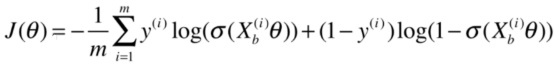逻辑回归损失函数的梯度：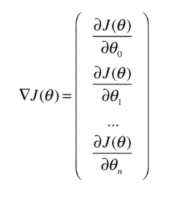计算预测函数Sigmoid函数的导数：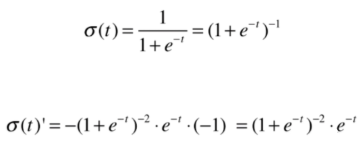代入：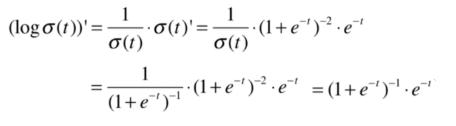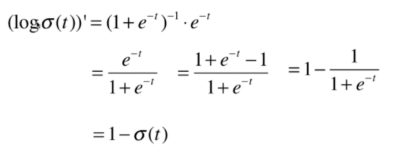计算过程：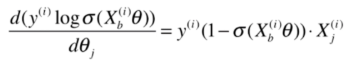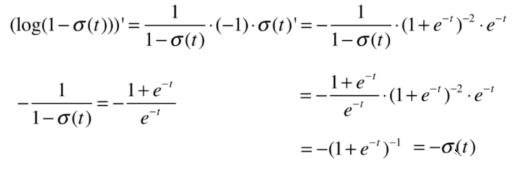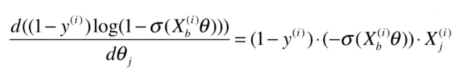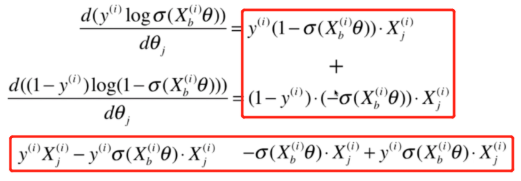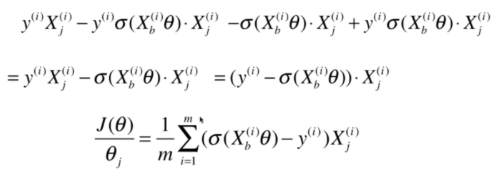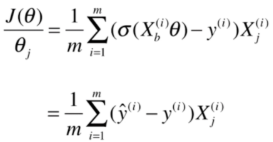最后得出逻辑回归损失函数的梯度：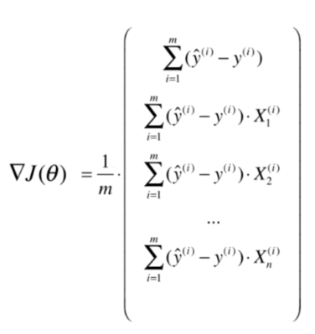回顾一下线性回归代价函数的梯度：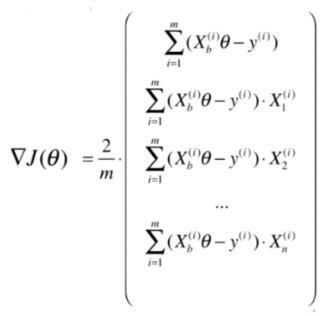对损失函数的梯度进行向量化：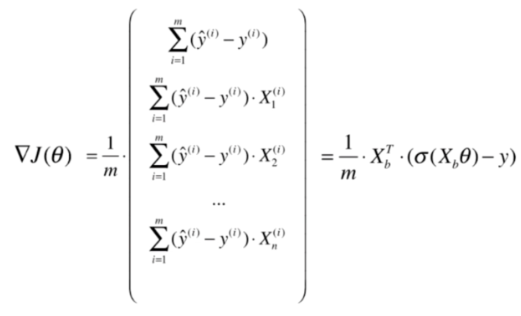回顾一下线性回归代价函数的梯度的向量化：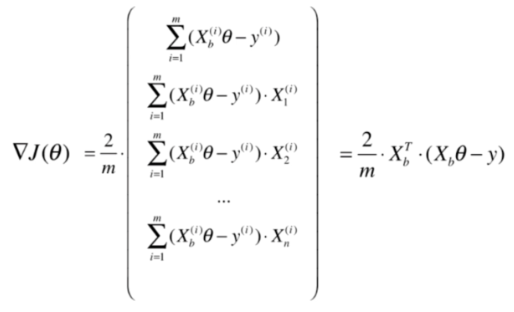参考资料：bobo老师机器学习教程


展开全文机器学习 python
• 线性回归和逻辑回归损失函数推导 @(数据挖掘) 线性回归和逻辑回归损失函数推导 一、线性回归最小二乘loss推导 二、logistics回归加sigmoid原因以及交叉熵损失函数推导 一、线性回归最小二乘loss推导 ...
线性回归和逻辑回归损失函数推导

@(数据挖掘)

线性回归和逻辑回归损失函数推导
一、线性回归最小二乘loss推导
二、logistics回归加sigmoid原因以及交叉熵损失函数推导

一、线性回归最小二乘loss推导

我们都知道线性回归是机器学习中最简单，使用范围也很广的一个算法，经典且使用。而它的损失函数最小二乘损失，大家也很熟悉，但是为什么要用最小二乘loss呢？正文开始：
可以通过一系列假设，从概率的角度去说明为什么选最小二乘（按理说，我们有许多函数可以定义损失）。

假设：
y(i)=θTx(i)+ε(i)${y}^{\left(i\right)}={\theta }^{T}{x}^{\left(i\right)}+{\epsilon }^{\left(i\right)}$$y^{(i)} = \theta^Tx^{(i)} + \varepsilon^{(i)}$
ε(i)=error${\epsilon }^{\left(i\right)}=error$$\varepsilon^{(i)} = error$
这里的error也就是模型和实际数值之间的误差值
根据中心极限定理(许多独立随机变量组合会符合高斯分布)，我们可以接着假设误差项符合高斯分布：
ε(i)∼N(0,σ2)${\epsilon }^{\left(i\right)}\sim N\left(0,{\sigma }^{2}\right)$$\varepsilon^{(i)} \sim N(0, \sigma^2)$
即概率密度函数为
P(ε(i))=12π√σexp(−(ε(i))22σ2)$P\left({\epsilon }^{\left(i\right)}\right)=\frac{1}{\sqrt{2\pi }\sigma }exp\left(-\frac{\left({\epsilon }^{\left(i\right)}{\right)}^{2}}{2{\sigma }^{2}}\right)$$P(\varepsilon^{(i)}) = \frac{1}{\sqrt{2\pi}\sigma} exp(-\frac{(\varepsilon^{(i)})^2}{2\sigma^2})$
上述误差函数的概率密度函数服从高斯分布，则我们易知：
P(y(i)|x(i);θ)=12π√σexp(−(y(i)−θTx(i))22σ2)$P\left({y}^{\left(i\right)}|{x}^{\left(i\right)};\theta \right)=\frac{1}{\sqrt{2\pi }\sigma }exp\left(-\frac{{\left({y}^{\left(i\right)}-{\theta }^{T}{x}^{\left(i\right)}\right)}^{2}}{2{\sigma }^{2}}\right)$$P\left ( y^{(i)}|x^{(i)};\theta \right ) = \frac{1}{\sqrt{2\pi }\sigma }exp\left ( -\frac{\left ( y^{(i)}-\theta^Tx^{(i)} \right )^{2}}{2\sigma^2} \right )$
即：y(i)|x(i);θ∼N(θTx(i),σ2)${y}^{\left(i\right)}|{x}^{\left(i\right)};\theta \sim N\left({\theta }^{T}{x}^{\left(i\right)},{\sigma }^{2}\right)$$y^{(i)}|x^{(i)};\theta \sim N(\theta^Tx^{(i)}, \sigma^2)$
这里需要特别注意，我们不把θ$\theta$$\theta$认为是随机变量，而是有着我们未知的确定值，也就是把它看成我们需要去估计得到的值，也就是说上面的概率P(y(i)|x(i);θ)$P\left({y}^{\left(i\right)}|{x}^{\left(i\right)};\theta \right)$$P\left ( y^{(i)}|x^{(i)};\theta \right )$ 意思是以θ$\theta$$\theta$为参数时，给定x(i)${x}^{\left(i\right)}$$x^{(i)}$条件下y(i)${y}^{\left(i\right)}$$y^{(i)}$的条件概率分布
假设不同输入x(i)${x}^{\left(i\right)}$$x^{(i)}$对应误差项ε(i)${\epsilon }^{\left(i\right)}$$\varepsilon^{(i)}$是独立同分布(IID:Independently and Identically Distributed;意思是条件独立的，但是都服从同一均值方差的高斯分布)：
则我们的模型可以用概率模型定义为一个极大似然估计问题：

L(θ)=P(y⃗ |x;θ)=∏i=1mP(y(i)|x(i);θ)=∏i=1m12π‾‾‾√σexp(−(y(i)−θTx(i))22σ2)$L\left(\theta \right)=P\left(\stackrel{\to }{y}|x;\theta \right)=\prod _{i=1}^{m}P\left({y}^{\left(i\right)}|{x}^{\left(i\right)};\theta \right)=\prod _{i=1}^{m}\frac{1}{\sqrt{2\pi }\sigma }exp\left(-\frac{{\left({y}^{\left(i\right)}-{\theta }^{T}{x}^{\left(i\right)}\right)}^{2}}{2{\sigma }^{2}}\right)$ L(\theta) = P(\vec{y}|x;\theta) =  \prod_{i=1}^{m}P\left ( y^{(i)}|x^{(i)};\theta  \right ) = \prod_{i=1}^{m} \frac{1}{\sqrt{2\pi }\sigma }exp\left ( -\frac{\left ( y^{(i)}-\theta^Tx^{(i)} \right )^{2}}{2\sigma^2} \right )
4. 所以最后我们的寻找最优模型的参数θ$\theta$$\theta$问题变成了一个求上式关于θ$\theta$$\theta$的极大似然估计问题，为了方便计算，我们常常求对数似然，得到：
logL(θ)=log∏i=1m12π‾‾‾√σexp(−(y(i)−θTx(i))22σ2)=mlog12π‾‾‾√σ+∑i=1m−(y(i)−θTx(i))22σ2$logL\left(\theta \right)=log\prod _{i=1}^{m}\frac{1}{\sqrt{2\pi }\sigma }exp\left(-\frac{{\left({y}^{\left(i\right)}-{\theta }^{T}{x}^{\left(i\right)}\right)}^{2}}{2{\sigma }^{2}}\right)=mlog\frac{1}{\sqrt{2\pi }\sigma }+\sum _{i=1}^{m}-\frac{{\left({y}^{\left(i\right)}-{\theta }^{T}{x}^{\left(i\right)}\right)}^{2}}{2{\sigma }^{2}}$ logL(\theta) = log \prod_{i=1}^{m} \frac{1}{\sqrt{2\pi }\sigma }exp\left ( -\frac{\left ( y^{(i)}-\theta^Tx^{(i)} \right )^{2}}{2\sigma^2} \right ) = m log\frac{1}{\sqrt{2\pi }\sigma } + \sum_{i=1}^{m}-\frac{\left ( y^{(i)}-\theta^Tx^{(i)} \right )^{2}}{2\sigma^2}
所以从上式易知，最大化logL(θ)$logL\left(\theta \right)$$logL(\theta)$问题就相当于最小化∑mi=1(y(i)−θTx(i))22σ2$\sum _{i=1}^{m}\frac{{\left({y}^{\left(i\right)}-{\theta }^{T}{x}^{\left(i\right)}\right)}^{2}}{2{\sigma }^{2}}$$\sum_{i=1}^{m}\frac{\left ( y^{(i)}-\theta^Tx^{(i)} \right )^{2}}{2\sigma^2}$问题，也就是我们定义的loss

二、logistics回归加sigmoid原因以及交叉熵损失函数推导

我们知道，其实逻辑回归模型变成了分类问题，但只是在线性回归的基础上加上了一个sigmoid函数，那么问题来了：
1. 为什么我们要选择使用一个sigmoid函数？
大家可以移步参考知乎上的回答：https://www.zhihu.com/question/35322351
2. 交叉熵损失函数的推导
- 同线性回归，我们可以把我们的模型用概率表示：
P(y(i)=1|x(i);θ)=hθ(x(i))$P\left({y}^{\left(i\right)}=1|{x}^{\left(i\right)};\theta \right)={h}_{\theta }\left({x}^{\left(i\right)}\right)$$P(y^{(i)}=1|x^{(i)};\theta) = h_{\theta} (x^{(i)})$
P(y(i)=0|x(i);θ)=1−hθ(x(i))$P\left({y}^{\left(i\right)}=0|{x}^{\left(i\right)};\theta \right)=1-{h}_{\theta }\left({x}^{\left(i\right)}\right)$$P(y^{(i)}=0|x^{(i)};\theta) = 1 - h_{\theta} (x^{(i)})$
- 我们可以进一步把两式整合：
P(y(i)|x(i);θ)=hθ(x(i))y(i)(1−hθ(x(i)))(1−y(i))$P\left({y}^{\left(i\right)}|{x}^{\left(i\right)};\theta \right)={h}_{\theta }\left({x}^{\left(i\right)}{\right)}^{{y}^{\left(i\right)}}\left(1-{h}_{\theta }\left({x}^{\left(i\right)}\right){\right)}^{\left(1-{y}^{\left(i\right)}\right)}$$P(y^{(i)}|x^{(i)};\theta) = h_{\theta} (x^{(i)})^{y^{(i)}}(1 - h_{\theta} (x^{(i)}))^{(1-y^{(i)})}$
- 同样我们可以把模型最优问题看做是极大似然估计问题：
L(θ)=P(y⃗ |x;θ)=∏mi=1P(y(i)|x(i);θ)=∏mi=1hθ(x(i))y(i)(1−hθ(x(i)))(1−y(i))$L\left(\theta \right)=P\left(\stackrel{\to }{y}|x;\theta \right)=\prod _{i=1}^{m}P\left({y}^{\left(i\right)}|{x}^{\left(i\right)};\theta \right)=\prod _{i=1}^{m}{h}_{\theta }\left({x}^{\left(i\right)}{\right)}^{{y}^{\left(i\right)}}\left(1-{h}_{\theta }\left({x}^{\left(i\right)}\right){\right)}^{\left(1-{y}^{\left(i\right)}\right)}$$L(\theta) = P(\vec{y}|x;\theta) = \prod_{i=1}^{m}P(y^{(i)}|x^{(i)};\theta) = \prod_{i=1}^{m} h_{\theta} (x^{(i)})^{y^{(i)}}(1 - h_{\theta} (x^{(i)}))^{(1-y^{(i)})}$
- 还是去对数似然：
logL(θ)=∑mi=1y(i)loghθ(x(i))+(1−y(i))log(1−hθ(x(i)))$logL\left(\theta \right)=\sum _{i=1}^{m}{y}^{\left(i\right)}log{h}_{\theta }\left({x}^{\left(i\right)}\right)+\left(1-{y}^{\left(i\right)}\right)log\left(1-{h}_{\theta }\left({x}^{\left(i\right)}\right)\right)$$logL(\theta) = \sum_{i=1}^{m}y^{(i)}logh_{\theta} (x^{(i)}) + (1-y^{(i)})log(1 - h_{\theta} (x^{(i)}))$
则，得证交叉熵函数
展开全文线性回归原理
• 以单样本为例，逻辑回归损失函数 C=−yz+ln(1+ez)C=-yz+ln(1+e^{z})C=−yz+ln(1+ez) 其中z=wxz=wxz=wx ∂C∂z=−y+11+e−z={1,y=10,other&amp;amp;amp;nbsp;values \frac{\partial C}{\partial z}=-y+\frac{1}{1...
这篇文章的目的是要记录一下自己学习对数损失函数时的一些疑惑，以及自己对疑惑的理解。这部分理解主要参考了下面这篇博客
https://www.cnblogs.com/zhangchaoyang/articles/8157893.html
通常，一些书籍会直接告诉我们对数损失函数如下
$log(1+e^{-ywx}), \tag{1}$
也叫做逻辑回归损失函数。通常，这时候我们会回想逻辑回归的损失函数，大部分文章或书籍的结果如下
$-ylog(g(x))-(1-y)log(1-g(x))\tag{2},$
其中$g(x)=\frac{1}{1+e^{-z}},z=wx$。
通过观察，容易发现，这两个式子直观上非常不同，但其实本质是一样的。接下去解释它们为什么是一样的。思路比较简单：以(2)式出发，通过一系列推导转换得到(1)。
以单样本为例，目标变量$y$的取值是1或0时，对(2)式进行等价转化，得到逻辑回归损失函数为
$C=-yz+ln(1+e^{z}),\tag{3}$
对$z$求导得
$\frac{\partial C}{\partial z}=-y+\frac{1}{1+e^{-z}}=\left \{\begin{array}{cc} -\frac{1}{1+e^{z}}, &y = 1\\ \ \frac{1}{1+e^{-z}}, & y = 0 \end{array}\right.,$
上述情况是在$y$的取值是1和0时推导的公式，当$y$的取值是1和-1时，公式的形式也变得优美一些,如下：
$\frac{\partial C1}{\partial z}=\frac{-y}{1+e^{yz}}$
而由等式右边可以推导出原函数$C1$的表达式如下：
$C1=log(1+e^{-yz})，$
也就是我们常见的当$y$是1和-1时的二分类逻辑回归损失函数。
这只是我的一些理解，解释$y$在不同取值情况下的表达式，应该是有更直接的方法来阐述这件事情。如果有谁知道相关文章，希望可以告诉我，谢谢！


展开全文• 这里只推导逻辑回归损失公式。 假设函数 hθ(x)=11+e−θTx(假设函数)(假设函数)hθ(x)=11+e−θTx h_\theta(x) = \frac{1}{1+e^{-\theta^Tx}} \tag{假设函数} 用于二分类 p(x)={hθ(x),(1−hθ(x)),if&...LR 最大似然
• 逻辑回归损失函数为啥是交叉熵 我的另一篇博客：逻辑回归为什么使用对数损失函数 逻辑回归为啥不用平方损失 原因很简单：就是会出现梯度消失问题，至于为啥看下面的这个推导（我真是无语！），其他的真的没啥说的...
• 优点 实现简单； 分类时计算量非常小，速度很快，存储资源低；...逻辑回归的公式为： h(x)=11+e−(wTx+b) h(x) = \frac{1} {1 + e^{-(w^Tx+b)}} h(x)=1+e−(wTx+b)1​ 假设有N个样本，样本的标...机器学习
• 首先说什么是凸函数。对区间[a,b]上定义的函数f，若它对区间中任意两点x1和x2，均有f((x1+x2)/2)&...线性回归模型中的损失函数为平方差损失函数，其是凸函数逻辑回归中，损失函数为交叉熵损失函数，因为...
• 一、背景知识 0.矩阵的转置与共轭转置 1.矩阵的迹 2.矩阵元算的交换律 3.矩阵迹的求导 4.矩阵的二阶范数 二、求导
• 在学习逻辑回归的过程中，通过3D图像可以直接观察损失函数的收敛速度，对自行确定学习速率提供参考损失函数公式：Octave程序如下：tx = linspace(100,-30,1000); %θ和X看做一个共同参数ty = round(unifrnd(0,1,1,...
• 机器学习的损失函数是人为设计的，用于评判模型...其次，既然不同标准并不冲突，那使用最小二乘作为逻辑回归损失函数当然是可以，那这里为什么不用最小二乘而用最大似然呢？请看一下最小二乘作为损失函数的函数...
• 最小二乘法的误差符合正态分布，而逻辑回归的误差符合的是二项分布，所以不能用最小二乘法来作为损失函数，那么能够用最大似然预计来做。 从求最优解的角度来解释： 如果用最小二乘法，目标函数就是,是非凸的，不...机器学习 深度学习
• 上面代表单条特征，下面代表多条特征的损失函数 2.误差度量： 准确率：=TP+Tn/（TP+FP+FN+TN） 查准率/精确率=TP/(TP+FP) 举例：抓小偷，务必提高精确率，预测准确，让好人少进来 查全率/召回率=TP/(TP+FN)举例：...
• Q：为什么深度学习损失函数往往要求是凸函数？（此处凸函数是指下凸，国内和国外凸函数的标准不一样，是相反的，吴恩达的机器学习教程凸函数是指下凸） A：使用梯度下降法优化策略，如果损失函数是非凸的，最后...机器学习 深度学习
• 文章目录1.标签为{0,1}损失函数推导2.标签为{+1,-1}损失函数推导2.1 似然函数2.2 损失函数 1.标签为{0,1}损失函数推导 ...纵向逻辑回归的一篇论文中，当标签为{+1,-1}时候，损失函数为： 这样的好处是在纵向联合学机器学习
• y(1,0) 逻辑回归损失3. y(-1,-1) 逻辑回归损失公众号 1. 逻辑回归 逻辑回归使用回归手段来做分类问题(主要是二分类).它使用sigmoid函数减小预测范围,将线性回归的结果限定在(0,1)之间,并将其作为条件概率进行建模....
• 从信息论交叉熵的角度看softmax/逻辑回归损失
•机器学习
• 逻辑回归——分类算法 代价函数的寻找 sigmoid函数的非线性会导致损失函数变得非线性，无法找到全局最优值，所以需要进行损失函数替换。 代价函数的合理性分析 预测正确，损失较小；预测错误，损失较...
• 这里不由的会想，为什么要引入这样的...有没有发现上面损失函数与下面凸集定义的形式差不多，上面y是属于0到1，凸集的性质大家可以看看书。凸函数：f(（x1+x2）/2)&lt;(f(x1)+f(x2))/2定义Df为凸集，对于任意下...
• 逻辑回归函数一般用在分类问题上。实际上也可以用最小二乘，但是最小二乘得到的权重效果比较差。 如果用最小二乘法，目标函数就是差值的平方和,是非凸的，不容易求解，很容易陷入到局部最优。如果用最大似然估计，...
• 逻辑回归（Logistic Regression） 前面说到，对于给定的样本数据集X, y，我们需要找到参数theta，使得用这样的方式，可以最大程度获得样本...将预测函数代入逻辑回归损失函数： 此函数没有公式解，只能使用梯度下...机器学习 python
• 一、线性回归损失函数的两种解释线性回归的损失函数是平方损失函数，为什么使用平方的形式，参考：线性回归损失函数为什么要用平方形式，讲得很清楚。 在线性回归中，对于训练数据样本(xi,yi)(x_i,y_i)，我们有如下...线性回归
• ## 逻辑回归中的损失函数的解释

万次阅读 多人点赞 2018-06-05 18:19:11
1.Logistic Regression（逻辑回归逻辑回归是机器学习中的一个非常常见的模型， 逻辑回归模型其实仅在线性回归的基础上，套用了一个逻辑函数。...2.损失函数 为了训练逻辑回归模型的参数w和b需要一个代价函...
• 什么是逻辑回归 1、解决分类问题 2、将样本的特征和样本发生的概率联系起来（概率是个数，所以称为回归问题） 3、既可以看作回归算法，也可以看作...逻辑回归损失函数 没有公式解，只能使用梯度下降法求解 ...机器学习
• ## 逻辑回归：损失函数与梯度下降

万次阅读 多人点赞 2017-09-05 15:28:04
1 sigmoid函数 2 极大似然估计MLE与损失函数 3 梯度下降 4 另一种形式的损失函数及其梯度 ...所以一般使用sigmoid函数来拟合：g(z)=11+e−z(1)g(z)={\frac 1{1+e^{-z}}}\tag{1}具体应用到逻辑回归算法中：梯度下降...